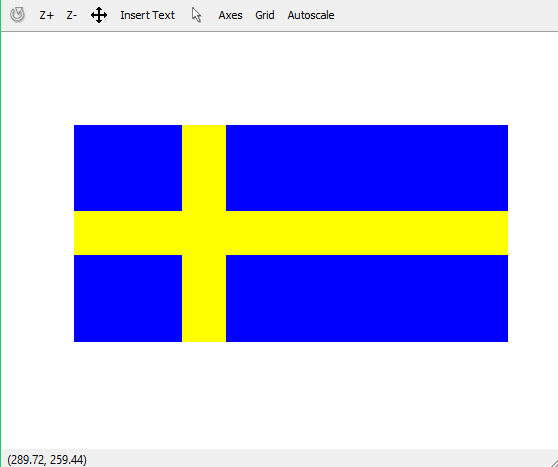# Draw Sweden Flag using matlab

A colored image can be represented as a 3 order matrix. The first order is for the rows, the second order is for the columns and the third order is for specifying the color of the corresponding pixel. Here we use the RGB color format, so the third order will take 3 values of Red, Green ans Blue respectively. The values of the rows and columns depends on the size of the image.

Prerequisite : RGB image representation

Approach :

• Make a 3 order zero matrix of dimensions 300X600X3. 300 denotes the number of pixels for rows, 600 denotes number of pixels for columns and 3 denotes the color coding in RGB format.
• Paint the complete image in blue. RGB code for blue is (0, 0, 255)
• Make the horizontal yellow bar. RGB code for yellow is (255, 255, 0)
• Make the vertical yellow bar.

Below is the implementation:

 `% matlab code to draw Sweden flag ` ` `  `I = zeros(300, 600, 3); ` ` `  `%painting the whole image blue ` `I(:, :, 3) = 255;  ` ` `  `%yellow bar ` `I(120:180, :, 1:2) = 255; I(120:180, :, 3) = 0; ` ` `  `%yellow column ` `I(:, 150:210, 1:2) = 255;I(:, 150:210, 3) = 0;  ` ` `  `%print the matrix as image ` `imshow(I)  `

Output:My Personal Notes arrow_drop_upCheck out this Author's contributed articles.

If you like GeeksforGeeks and would like to contribute, you can also write an article using contribute.geeksforgeeks.org or mail your article to contribute@geeksforgeeks.org. See your article appearing on the GeeksforGeeks main page and help other Geeks.

Please Improve this article if you find anything incorrect by clicking on the "Improve Article" button below.

Article Tags :

Be the First to upvote.

Please write to us at contribute@geeksforgeeks.org to report any issue with the above content.